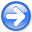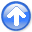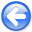Tomographic full waveform inversion and linear modeling of multiple scatteringNext: Multiple-scattering modeling Up: Conventional Full Waveform Inversion Previous: Gradient computation with FWI

## Problems with FWI

Unfortunately, high-order scattering must be taken into account to model accurately wavefield perturbations when the velocity perturbations have wide spatial extent and/or large amplitude. Such velocity perturbations cause significant (larger than one fourth of wave cycle) time shifts in the propagating wavefield. The linear operator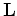cannot model large time shifts because the source function on the right-hand side of equation 8 is triggered by the background wavefield reaching a velocity perturbation, and consequently it has the timing as the background wavefield. Furthermore, the perturbed wavefield is propagated with the background velocity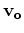. In mathematical terms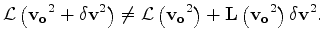(9)

The problem is even deeper. Whencauses large time shifts by multiple scattering, there is no perturbation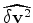that can model those time shifts by single scattering; that is,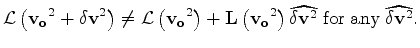(10)

The non linearity of the modeling operator makes the objective function equation 2 to be non convex when the velocity perturbations are sufficiently large. Figure 1 shows an example of non-convexity of the objective function. The result correspond to several 1D transmission problems sharing the same starting velocity (1.2 km/s) and with different true velocities. For all these experiments the source-receiver offset is 4 km and the source function is a zero-phase wavelet bandlimited between 5 and 20 Hz. The FWI norm is plotted as a function of the true velocity. If the true velocity is lower thankm/s or larger than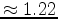km/s a gradient based method will not converge to the right solution, even in this simple and low-dimensionality example.

The challenges of solving the optimization problem in equation 1 by gradient based optimization can be alternatively represented by graphing, as a function of the initial velocity error, the search direction (opposite sign of the gradient direction) of the objective function with respect to velocity square. Figure 2 display this function computed by applying the adjoint of the linear operatorto the data residuals; that is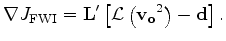(11)

For a gradient based method to converge, the search direction should be always negative when the true velocity is lower than 1.2 km/s, and positive when the true velocity is higher.Tomographic full waveform inversion and linear modeling of multiple scatteringNext: Multiple-scattering modeling Up: Conventional Full Waveform Inversion Previous: Gradient computation with FWI

2012-10-29